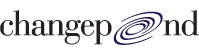# Placement Papers - ChangepondCHANGEPOND PAPER
Posted by :
Laxman majjiga
(17)
There were two sections Aptitude & Technical

Aptitude

1.If m-4 is 7 more than n then m+9 is how much more than n+2

2.If 10x coins are added to the no. of original coins he has (5y + 1) times more coins. Find out how many coins he had originally in terms of x and y

3. Find out the Simple Interest paid for a sum of \$4000 at the rate of 8% per annum for 3 months.

4. If it takes 10 technicians working 6 hours to build a server. They start woring at 11 AM and 1 technician is added per hour starting at 5 PM. At what time they will finish the server?

5. A chart was there giving the speed of train after some minutes like
0 30 60 90 120 150 180
40 45 50 55 60 65 70

6. Find the % increase in the speed of train?

7. If it is extrapolated further what will be the speed after 5 hrs.

8. If the data is for 10 bogies and for every 2 bogies added speed decreases by 5% what will be the speed after 5 hrs.

9.The type of questions where Both stmt are necessary First one is sufficient in itself.. What is the perimeter of the quadrilateral?

11.The area of the quadrilateral is 36 What is a*b

12.6a+4b=43
3a+12b=63

13. The house is big and lovely but since nobody uses it it is pretty much a white Elephant What does White Elephant mean?

14. Sometime they irritate me because they are up with the lark whereas I like to lie in What does Up with Lark mean?

Technical

1. What exception can an overridden method throw in comparison with the method it is overriding?

2. What does parseQueryString return?

3. Anonymous classes Can they have constructors?

4. what is wrong with this
abstract class MyClass
{
transient a,
synchronized b.

5.How can an inner class access the members of outer class?

6.Find the output:
String a=abc;
String b=abc;
If(a==b)
System.out.println(1);
Else
System.out.println(2);
If a.equals(b)
System.out.println(3);
Else
System.out.println(4);

7. Find the output Code showing Call by reference and call by value was given Array was used.

8. Some For stmts was given Mark all valid once

9. byte b=1
While(++b > 0);
System.out.println(b);